# How to Use Excel’s INDEX/MATCH Formula With Multiple Criteria

How to Use Excel’s INDEX/MATCH Formula With Multiple Criteria

Excel is an incredibly powerful software – if you know how to leverage it. With so many functions and formula options, there’s something new to learn every day.

The INDEX/MATCH formula can help you find data points quickly without having to manually search for them and risk making mistakes.

Let’s dive into how that formula works and review some helpful use cases.

## Understanding INDEX and MATCH Functions Individually

Before you can understand how to use the INDEX and match formula, it’s valuable to know how each function works on its own. That will offer some clarity on how both work together once combined.

The INDEX function returns a value or the reference to a value within a table or range based on the rows and columns you specify. Think of this function as a GPS – it helps you find data within a document but first, you need to narrow down the search area using rows and columns.

The MATCH function identifies a specific item in a range of cells then returns the relative position of that item in the range or the exact match.

For instance, say the range A1:A4 contains the values 15, 28, 49, 90. You want to know how the number “49” is relative to all values within the range. You would write the formula =MATCH(49,A1:A4,0) and it would return the number 3 because it’s the third number in the range. The 0 in the formula represents “exact match.”

Now that we’ve got the basics out of the way, let’s get into how to combine the formula and use it for multiple criteria.

## How to Use the INDEX and MATCH Formula with Multiple Criteria

The formula for the INDEX/MATCH formula is as follows: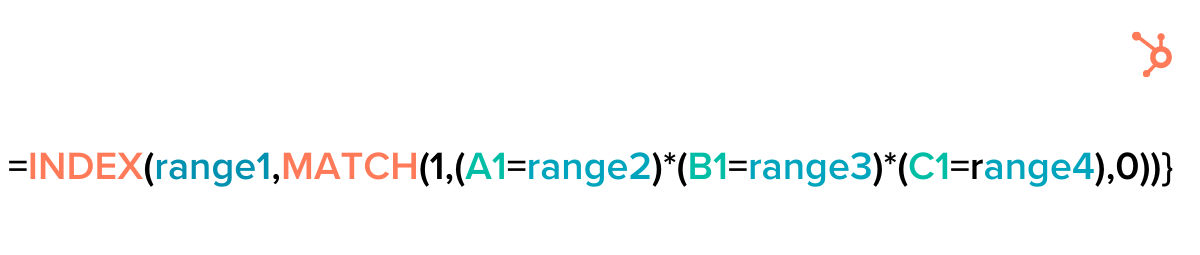Here’s how each function works together: Match finds a value and gives you its location. It then feeds that information to the INDEX function, which turns that information into a result.

To see it in action, let’s use an example.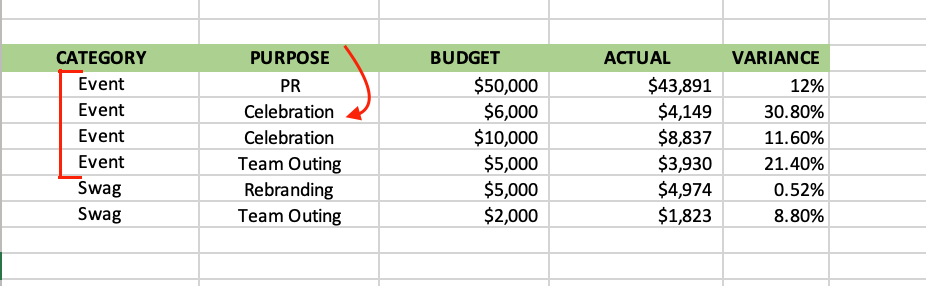This Excel sheet features a marketing budget for two categories: Events and company swag gifts. There are four purposes: Public relations (PR), celebration, team outing, and rebranding. The sheet also includes the defined budget and the actual expense for each category.

This is where the INDEX and MATCH formula comes in handy when using it for multiple criteria. You can quickly find the answer(s) you’re looking for and limit mistakes that would happen when searching manually.

Say you want to know the variance for an event that had a purpose of celebration with a budget of 10,000 – here’s how you’d do it.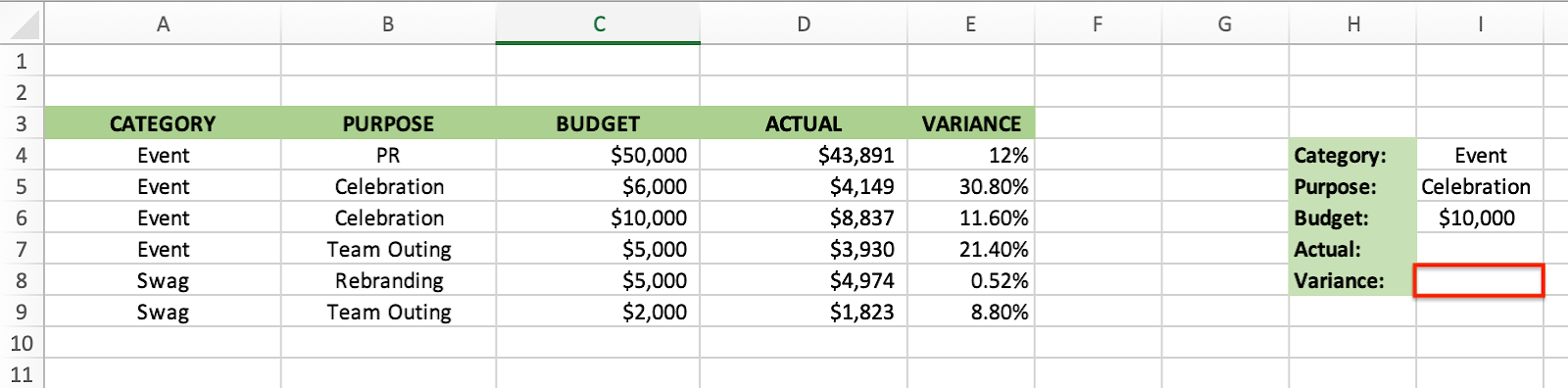First, take note of the row numbers and columns. The answer you’re looking for will go in I8. Here’s how the formula will look: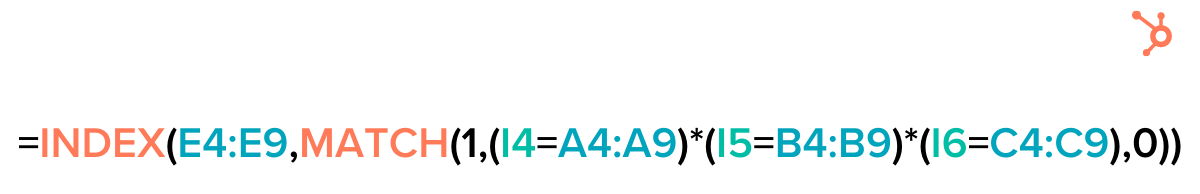Let’s break down how you get there.

### 1. Create a separate section to write out your criteria.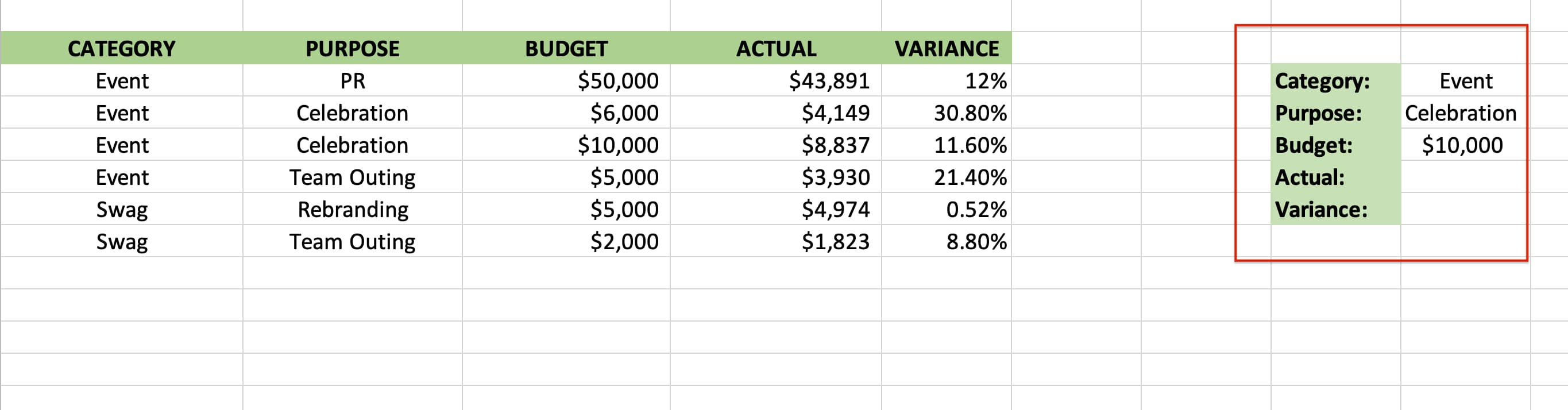The first step in this process is by listing out your criteria and the figure you’re looking for somewhere in your sheet. You’ll need this section later to create your formula.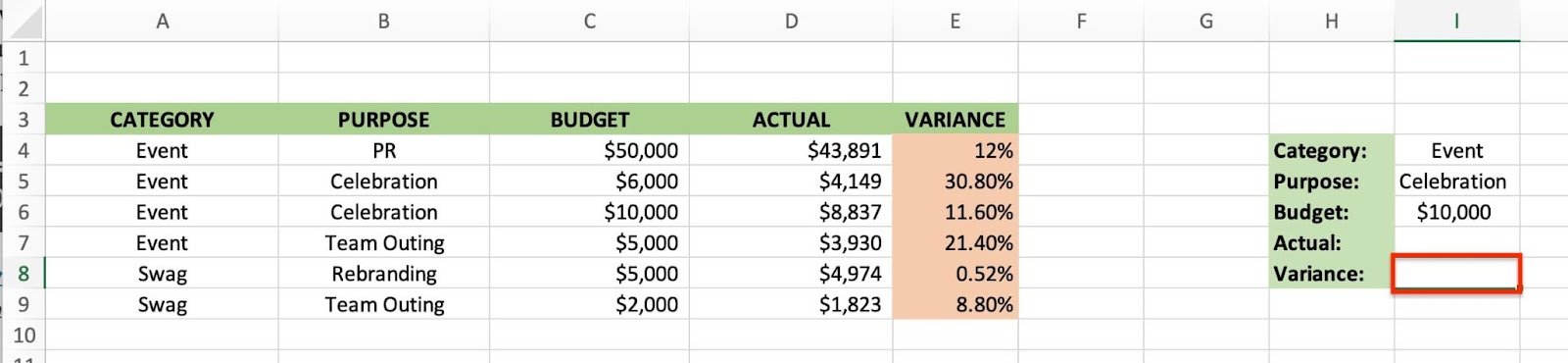The formula starts with your GPS, which is the INDEX function. You’re looking for the variance, so you select rows E4 through E9, as that is where the answer will be.

The more columns you have, the more ranges you’ll need to add to narrow down your results.

As a reminder, you’re looking for the variance for an event that had a \$10,000 budget and had a purpose of celebration. This means that you’ll have to tell Excel which rows hold the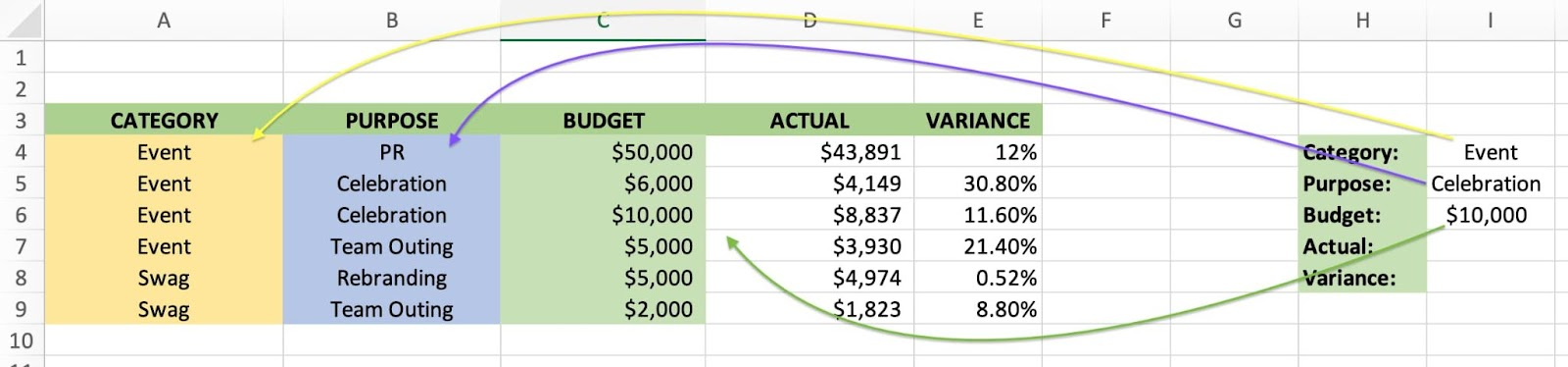Starting with the “event,” criteria, you find it first in I4., with its range located in column A between rows 4 and 9.

Follow the same process for “celebration” – it’s in I5 and its range is B4 and B9. Lastly, the “\$10,000” is in I6, with a range of C4 through C9.

The last step here is to add 0, which means you’re looking for an exact match.

That’s how you end up with this final formula:### 4. Run the formula.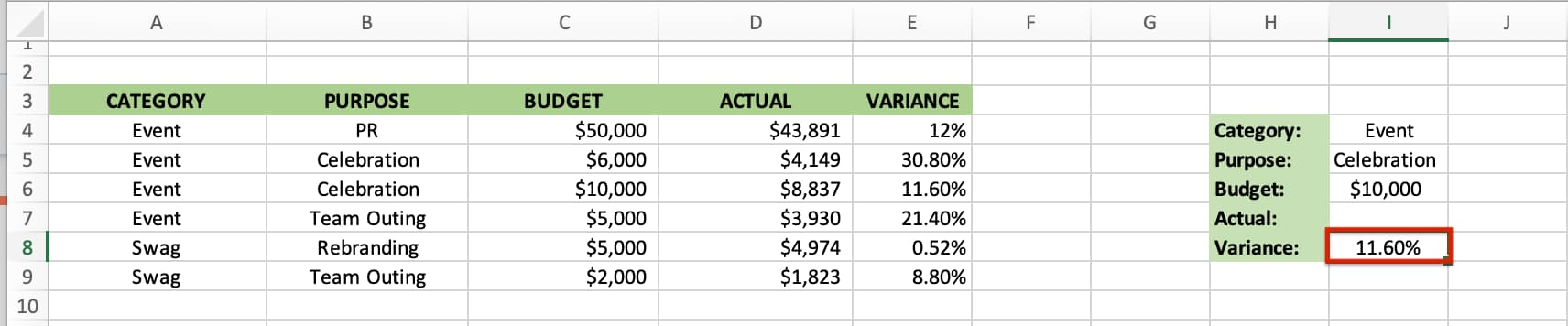Because this is an array formula, you must press Ctrl+Shift+Enter to get the right results, unless you are using Excel 365.

There you have it!Go to Source